Next: Non-spatial with Class Proportions Up: Continuous Weights Mixture Model Previous: Continuous Weights Mixture Model

### Non-spatial without Class Proportions

We are going to approximate the distribution in equation 3 by replacing the discrete labels,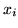, with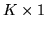continuous weights vectors,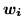: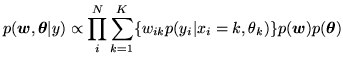(9)

where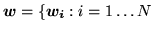} and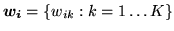is the continuous weights vector at voxel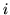. Equation 9 only approximates equation 3 if we apply certain constraints to the continuous weights vectors. If we choose a prior on the continuous weights vector,, with the constraints that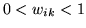and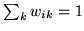, then as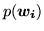tends to delta functions at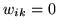and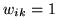, then equation 9 will tend to equation 3. Therefore, to apply these constraints the prior we use is: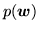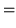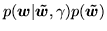(10)

where: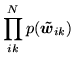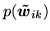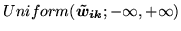(11)

and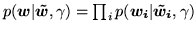, where crucially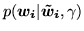is a deterministic relationship by whichand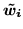are related by the logistic transform: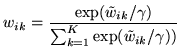(12)

The normalising constant in the logistic transform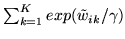ensures that the conditionis met. This expression also ensures that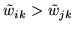, if and only if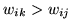. Figure 1 shows how the logistic transform produces an approximation to the delta functions asgets smaller. We fix the value ofto 0.05 whilst bounding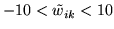, this ensures that we get the desired approximation to delta functions at 0 and 1, whilst ensuring that we can compute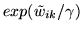without causing overflow.

To summarise, we now have two vectors of continuous weights at each voxel,and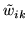.are weights which have a prior on them which is uniform on the real line. We then use the logistic transform to deterministically map the weightstoat each voxel. Then,are the continuous weights which represent approximations to the discrete labels with delta functions at 0 and 1.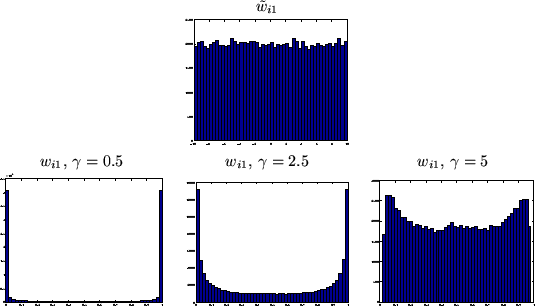Next: Non-spatial with Class Proportions Up: Continuous Weights Mixture Model Previous: Continuous Weights Mixture Model